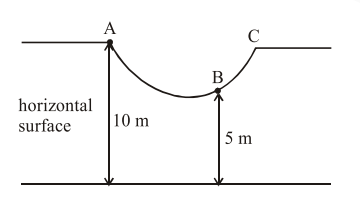# As shown in the figure, a particle of mass 10

Question:

As shown in the figure, a particle of mass 10 $\mathrm{kg}$ is placed at a point A. When the particle is slightly displaced to its right, it starts moving and reaches the point $B$. The speed of the particle at $\mathrm{B}$ is $\times \mathrm{m} / \mathrm{s}$. (Take $\mathrm{g}=10 \mathrm{~m} / \mathrm{s}^{2}$ )

The value of ' $x$ ' to the nearest integer is_________.Solution:

Using work energy theorem,

$\mathrm{W}_{\mathrm{g}}=\Delta \mathrm{K} . \mathrm{E}$

$(10)(g)(5)=\frac{1}{2}(10) v^{2}-0$

$\mathrm{v}=10 \mathrm{~m} / \mathrm{s}$